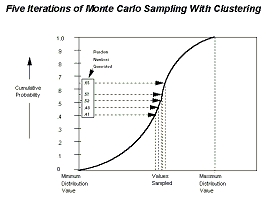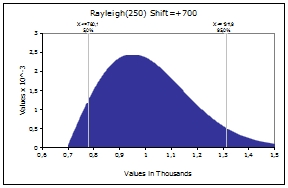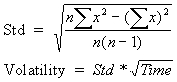# Glossary: Statistics

Certain or Uncertain

You may know the values your variables will take in the time frame of your model — they are certain, or what statisticians call “deterministic”. Conversely, you may not know the values they will take — they are uncertain, or “stochastic”. If your variables are uncertain you will need to describe the nature of their uncertainty. This is done with probability distributions, which give both the range of values that the variable could take (minimum to maximum), and the likelihood of occurrence of each value within the range.

Correlation

Correlation is a quantitative measurement of the strength of a relationship between two variables. The most common type of correlation is linear correlation, which measures the linear relationship between two variables. The rank order correlation value can vary between -1 and 1. A value of 0 indicates there is no correlation between variables; they are independent. A value of 1 indicates a complete positive correlation between the two variables; when the input value samples “high,” the output value will sample “high.” A value of -1 indicates a complete inverse correlation between the two variables; when the input value samples “high,” the output value will sample “low.” Other correlation values indicate a partial correlation; the output is affected by changes in the selected input, but may be affected by other variables as well.

Deterministic

The term deterministic indicates that there is no uncertainty associated with a given value or variable.

Independent or Dependent

In addition to being certain or uncertain, variables in a Risk Analysis model can be either “independent” or “dependent”. An independent variable is totally unaffected by any other variable within your model. For example, if you had a financial model evaluating the profitability of an agricultural crop, you might include an uncertain variable called Amount of Rainfall. It is reasonable to assume that other variables in your model such as Crop Price and Fertilizer Cost would have no effect on the amount of rain — Amount of Rainfall is an independent variable. A dependent variable, in contrast, is determined in full or in part by one or more other variables in your model. For example, a variable called Crop Yield in the above model should be expected to depend on the independent variable Amount of Rainfall. If there’s too little or too much rain, then the crop yield is low. If there’s an amount of rain that is about normal, then the crop yield would be anywhere from below average to well above average. Maybe there are other variables that affect Crop Yield such as Temperature, Loss to Insects, etc.

Mean/average

The mean or average of a set of values is the sum of all the values in the set divided by the total number of values in the set; or the average value of the set.

Monte Carlo sampling

Monte Carlo sampling refers to the traditional technique for using random or pseudo-random numbers to sample from a probability distribution. The term Monte Carlo was introduced during World War II as a code name for simulation of problems associated with development of the atomic bomb. Today, Monte Carlo techniques are applied to a wide variety of complex problems involving random behaviour. A wide variety of algorithms are available for generating random samples from different types of probability distributions.

Monte Carlo sampling techniques are entirely random — that is, any given sample may fall anywhere within the range of the input distribution. Samples, of course, are more likely to be drawn in areas of the distribution, which have higher probabilities of occurrence. In the cumulative distribution shown earlier, each Monte Carlo sample uses a new random number between 0 and 1. With enough iterations, Monte Carlo sampling “recreates” the input distributions through sampling. A problem of clustering, however, arises when a small number of iterations are performed.In the illustration shown here, each of the 5 samples drawn falls in the middle of the distribution. The values in the outer ranges of the distribution are not represented in the samples and thus their impact on your results is not included in your simulation output.

Clustering becomes especially pronounced when a distribution includes low probability outcomes, which could have a major impact on your results. It is important to include the effects of these low probability outcomes. To do this, these outcomes must be sampled. But, if their probability is low enough, a small number of Monte Carlo iterations may not sample sufficient quantities of these outcomes to accurately represent their probability.

Skewed distributionSkewness is a measure of the shape of a distribution. Skewness indicates the degree of asymmetry in a distribution. Skewed distributions have more values to one side of the peak or most likely value — one tail is much longer than the other is. A skewness of 0 indicates a symmetric distribution, while a negative skewness means the distribution is skewed to the left. Positive skewness indicates a skew to the right.

This distribution is skewed to the right, indicating upside potential rather that downside risk.

Standard deviation

The standard deviation is a measure of how widely dispersed the values are in a distribution or how much they deviate – on average – from the mean or average value. Equals the square root of the variance.

Stochastic

Stochastic is a synonym for uncertain, risky.

Value @ Risk

Anybody who owns a portfolio of investments knows there is a great deal of uncertainty about the future worth of the portfolio. Recently the concept of value at risk (VaR) has been used to help describe a portfolio’s uncertainty. Simply stated, value at risk of a portfolio at a future point in time is usually considered to be the fifth percentile of the loss in the portfolio’s value at that point in time. In short, there is considered to be only one chance in 20 that the portfolio’s loss will exceed the VAR. To illustrate the idea, suppose a portfolio today is worth \$100. We simulate the portfolio’s value one year from now and find there is a 5% chance that the portfolio’s value will be \$80 or less. Then the portfolio’s VaR is \$20 or 20%.

Conficence levels:

68,0 % Std.dev.*1
90,0 % Std.dev.*1,65
95,0 % Std.dev.*2
99,7 % Std.dev.*3

Variance

The variance is a measure of how widely dispersed the values are in a distribution, and thus is an indication of the “risk” of the distribution. It is calculated as the average of the squared deviations about the mean. The variance gives disproportionate weight to “outliers”, values that are far away from the mean. The variance is the square of the standard deviation.

Volatility

Volatility can be measured as the Standard deviation * square root of time, orTop